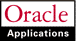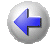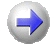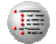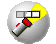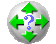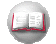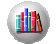Previous Next Contents Index Navigation Glossary Library

## Expenditure Types

When you define an expenditure type, you assign it a unit, an expenditure category, a revenue category, and one or more expenditure type classes.

You also specify whether an expenditure type requires a cost rate. An expenditure type with the following attributes requires a cost rate:

• A unit other than dollars
• A non-labor expenditure type class
See: Cost Rates.

For supplier invoice expenditure types, if you specify that a rate is required, Oracle Projects requires you to enter a quantity in Oracle Payables for invoice distributions using that expenditure type. When you interface the invoice distribution to Oracle Projects, Oracle Projects copies the quantity and amount to the expenditure item and calculates the rate. If you define a supplier invoice expenditure type with the Rate Required option disabled, then the quantity of the expenditure item is set to the amount you enter in Oracle Payables.

### Multiple Expenditure Type Classes Per Expenditure Type

You can assign multiple expenditure type classes to an expenditure type. For example, an expenditure with the expenditure type Materials can have the expenditure type class Supplier Invoice if it originated in Oracle Payables, and the expenditure type class Inventory if it originated in Oracle Inventory. This example is illustrated below:

 Expenditure Type Module Where Expenditure Originated Expenditure Type Class Materials Oracle Payables Supplier Invoice Materials Oracle Inventory Inventory Table 1 - 81. (Page 1 of 1) Example of Multiple Expenditure Type Classes for One Expenditure Type

This feature allows you to use a single expenditure type to classify as many different costs as you require. You can use the same expenditure type for expenditures that have different origins (and therefore different accounting), but which should otherwise be grouped together for costing, budgeting, or summarization purposes.

See: Defining Expenditure Types

Previous  Next          Contents  Index  Navigation  Glossary  Library